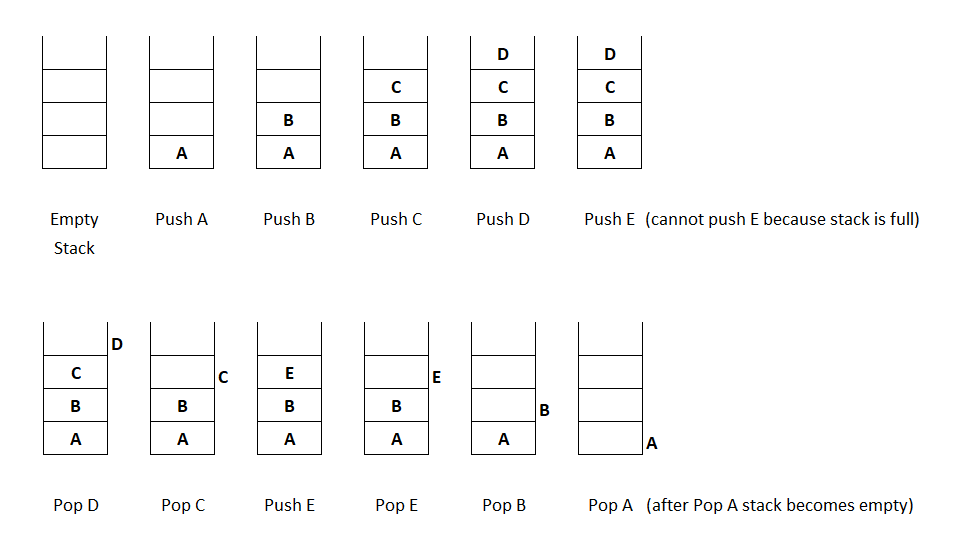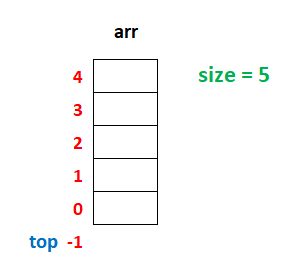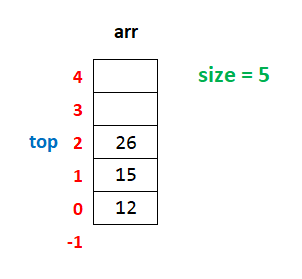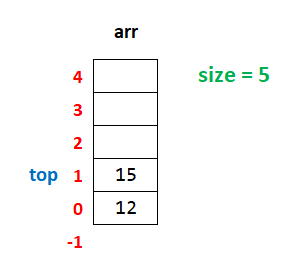# Stack in C Programming

###### Data Structure in C

In this lesson, we will understand what is Stack in C Programming and how to create them along with some examples.

## What is Stack in C

A Stack in C is a data structure in which we can insert or delete element only at the top of the stack.

We can see the example of a stack in our daily life as the stack of books.In the above image, we can see that we can take a book only from the top. Similarly, we can keep one more book only at the top.## Operation on Stack

There are two operations possible on the stack.

• Push - When we add an element in the stack.
• Pop - When we delete an element from the stack.

To understand how the above operations work on a stack. See the example given below.From the above image, we can see that the elements, which are added (push) last in the stack is deleted (pop) first from the stack.

The stack behaves like a last in first out manner. It means that when an element is added to a stack, it becomes the last element unless we add more and, when we remove an element from the stack, the last added element removed first.

So a stack is also known as LIFO (Last In First Out) data structure.

## Implementation of Stack in C

The stack in C programming can be implemented in two ways using:

• Array

In this lesson, we will see the implementation of a stack using an array. We will also discuss stack using a single linked list later on in the subsequent lesson.

## Array Implementation of Stack

Since a stack is a collection of the same type of elements, so we can implement the stack using an array.In the above image, we can see an array named arr in a vertical manner whose size is 5. We take a variable top, which keeps the position of the top element in the array.

### Push Operation on Stack

For push operation on the stack first, we check if the value of top is equal to the value of size-1 then, we will display a message Stack is full, else we will increase the value of top by 1 and add the element in the array at the new location of top.

#### Example

``````if(top==size-1)
{
printf("Stack is full\n");
}
else
{
top=top+1;
arr[top]=new_item;
}``````

If we insert three elements, say 12, 15 and 26 to the stack, then the stack will look like as shown in the image below.### Pop Operation on Stack

For pop operation on the stack first, we check if the value of top is equal to -1 then, we will display a message Stack is empty, else we will display the popped element on the screen and then decrease the value of top by 1.

#### Example

``````if(top==-1)
{
printf("Stack is empty\n");
}
else
{
printf("Element Popped = %d",arr[top]);
top=top-1;
}``````

If we delete the last elements 26 from the stack, then the stack will look like as shown in the image below.## Program of Stack using Array

Below is the complete program of stack in C using an array having size 5.

#### Stack Program in C using Array

``````#include <stdio.h>
#include <conio.h>
#include <stdlib.h>
#define size 5

int main()
{
int arr[size],top=-1,ch,n,i;

for(;;)		// An infinite loop
{
system("cls");		// for clearing the screen
printf("1. Push\n");
printf("2. Pop\n");
printf("3. Display\n");
printf("4. Exit\n");
printf("Enter Choice: ");
scanf("%d",&ch);

switch(ch)
{
case 1:
if(top==size-1)
{
printf("Stack is full");
getch();	// pause the loop to see the message
}
else
{
printf("Enter a number ");
scanf("%d",&n);
top++;
arr[top]=n;
}
break;

case 2:
if(top==-1)
{
printf("Stack is empty");
getch();	// pause the loop to see the message
}
else
{
printf("Number Popped = %d",arr[top]);
top--;
getch();	// pause the loop to see the number
}
break;

case 3:
if(top==-1)
{
printf("Stack is empty");
getch();	// pause the loop to see the message
}
else
{
for(i=top; i>=0; i--)
{
printf("%d\n",arr[i]);
}
getch();	// pause the loop to see the numbers
}
break;

case 4:
exit(0);
break;

default:
printf("Wrong Choice");
getch();	// pause the loop to see the message
}
}
return 0;
}``````

In the above program, we have defined a macro named size having value 5 using the statement #define. We can use the word size to declare the size of the array as int arr[size]. When we run the above program, the word size will be replaced by its value 5. So the size of the array will be 5.